## General Mechanical

•Md Ahad
Subscriber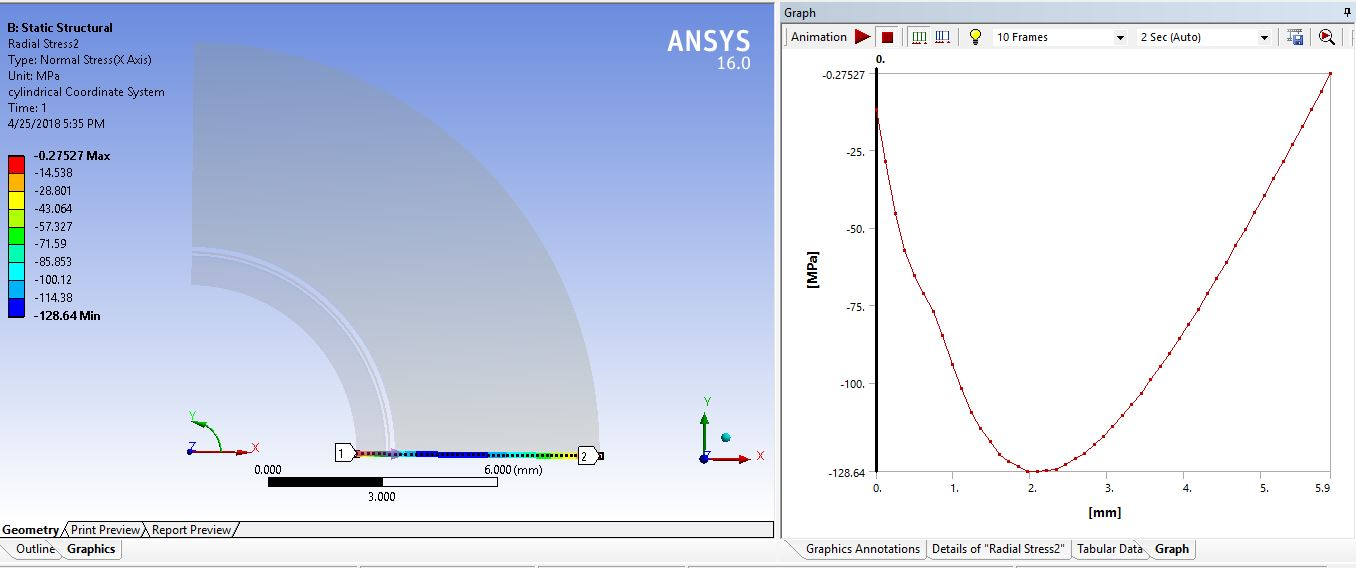How can i get Residual radial stress at room temperature, after the 1000 C temperature was applied and removed in a plane stress or plane strain 2D cylinder. I can get the radial stresses only but don't know how to get the residual radial stress from the post processing. I have attached the archive file for better understanding to help.

•peteroznewman
Subscriber

There are two ways to get residual stress in your problem.

One is where different materials are bonded at 1000 C without stress, then cooled to room temperature (22 C) and elastic residual stress is created due to the difference in CTE between the materials. If the material were reheated to 1000 C, the stress would again be zero. If this is your case, please confirm.

Another way is when the different materials were bonded at room temperature, so have no residual stress at 22 C, but at least one material passes its yield point as the temperature is raised to 1000 C and lowered again. This could result in permanent plastic deformation (or crushing if a porous material) and there would be a residual stress when the material returns to room temperature. If this is your case, your model has no material properties for plasticity or any nonlinear behavior.

A model could have both elastic and plastic causes of residual stress, so even if it was bonded at 1000 C, one material could pass its yield point as it cools to 22 C, which would be a different model and result from the first case.

Regards

•Md Ahad
Subscriber

Sir peteroznewman,

First of all I am very happy to see your reply.I have been always looking for you when i am getting some mechanical workbench problem.I would like to learn the both ways to get the residual stresses sir.

If i provide the adhesive strength and,Hardness,Toughness and melting point then will it fulfill the demand for plastic properties?

Another thing i can give the numerical solution of the graph (deformation vs distance) of this model.If it helps you to consider the conditions and others on.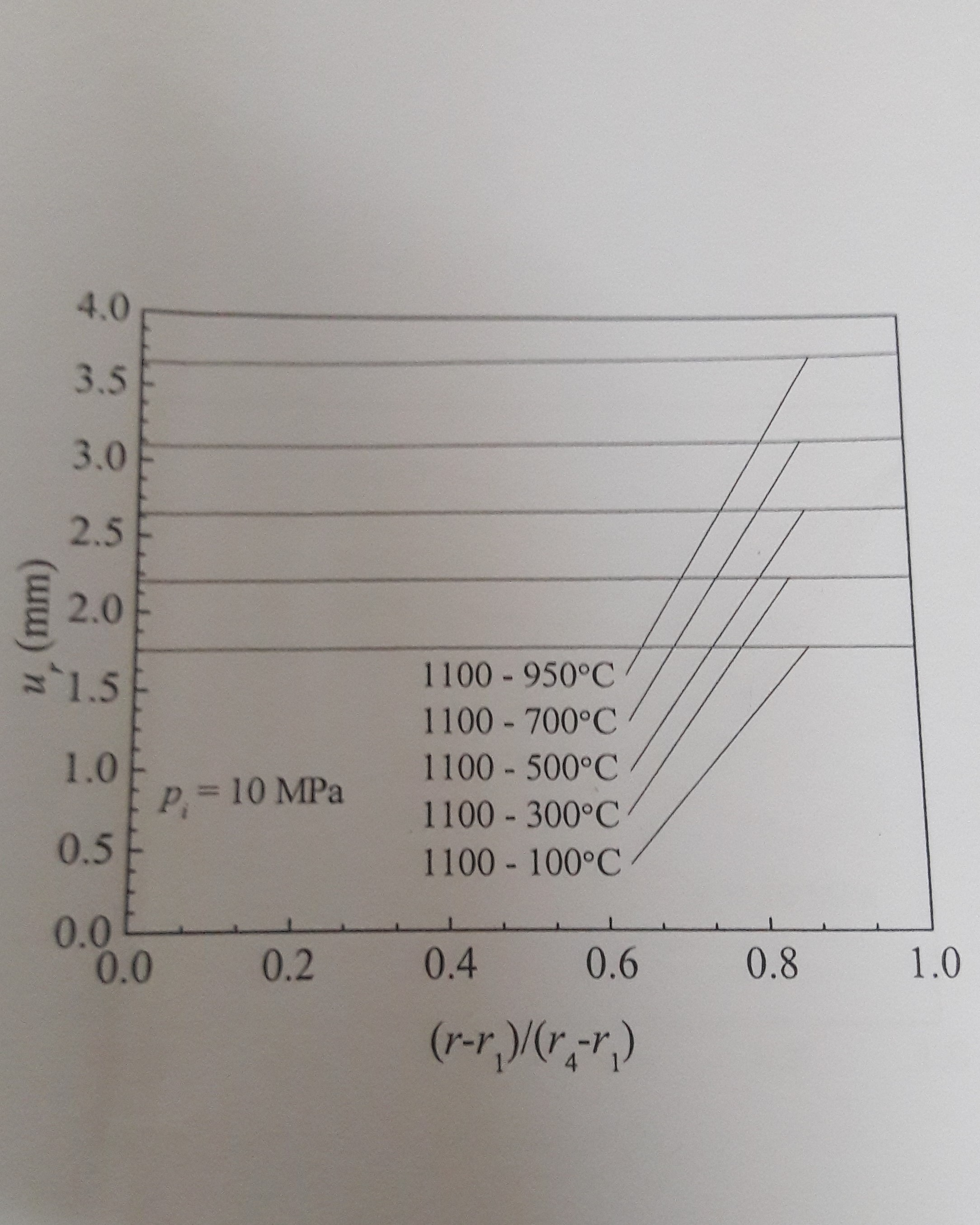This is the graph of deformation.So we can justify our assumptions by checking the graph of our simulated result with this graph too.The line 1100-100 means the inner line gets 1100*c temperature and the outer line of the cylinder gets 100*c temperature in all the graphs i have provided you so far.Will our model represent the similar graph of deformation sir? I have checked but i got a non linear curve line going upwards.

Sir you can take your time to help.I have bothered you much.Very Sorry for that.

Regards

•Md Ahad
Subscriber

Dear peteroznewman,

Regards.

•peteroznewman
Subscriber

If i provide the adhesive strength and,Hardness,Toughness and melting point then will it fulfill the demand for plastic properties?

The simplest way to create a material model that includes plasticity is to know the yield strength. With that you can add Bilinear Kinematic Hardening plasticity to a material that already has Isotropic Elasticity. Bilinear Kinematic Hardening requires one other value, the Tangent Modulus. This value describes the post-yield hardening behavior. If the Tangent Modulus is unknown, then a conservative approach is to set the Tangent Modulus to a value of zero. This creates what is known in textbooks as an Elastic Perfectly Plastic material.

Yield strength may be a function of temperature and you can input a table of temperature and yield strength. These might go up to the softening temperature, but at the melting temperature, the material is no longer a solid, but a liquid so there is no longer an elastic response to stress, there is a flow that is characterized by a material property called viscosity.

I can give the numerical solution of the graph (deformation vs distance) of this model.

When you say "this model", do you mean the model in the book that has the graph you are trying to match?  Yes, the tabular data of the output of the book model would be useful for adjusting parameters in an ANSYS model to try to match it.  However, what would be far more useful is the full description of the book model. A full description would be the geometry of all the layers, the material properties of all the layers, the temperature at which the layers are bonded together, the load history of the assembly and the conditions at the time the graph is plotted.

•Md Ahad
Subscriber

I have attached the model i tried last time.

Put 10MPa pressure on the inner line of the cylinder and inner line temperature 1100 and outer line temperature 100.

And then teach me how can i get the residual stresses , deformation graph(like the above attached photo already).

•peteroznewman
Subscriber

Here is a graph of radial displacement from your model in red averaging about 0.024 mm, while the value of the line for the 1000-100 condition that I read off the u plot from the book above is about 1.7 mm and I plotted that with a green line. The green line is a factor of about 70 times larger than the red line.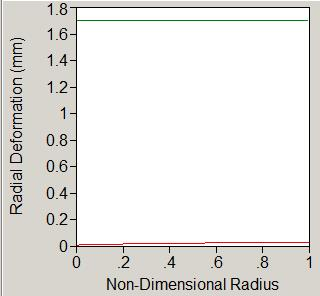The enormous difference between the value of the plot in the book and the value in your model is conclusive evidence that your model does not reproduce the physics that are in the book.

If you are interested in reproducing the results in the book with an ANSYS model, then you have to show for the numerical model in the book all of the following: the mathematical model, the assumptions made by the mathematical model, the inputs to the numerical model, the time-history of the materials in the numerical model.  Where does it say in the book that residual stresses are being plotted in the stress plot? I asked about the temperature during bonding of the layers. Where is that information?

•Md Ahad
Subscriber

Thank you very much.I have understood you.

I will study what you have given me the doubts.Give some time to find out the answer of your demand.I will try to give the whole information what you asked me.

•Md Ahad
Subscriber

Dear sir,

Can you please show me how to produce this graph from my model?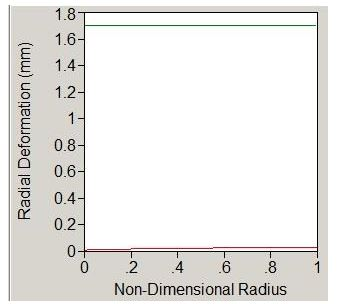And sir i will know you the other information as soon as possible.I am studying about your doubts.

•peteroznewman
Subscriber

I used JMP to make the plot, but if you have Excel, you can make a similar plot.  Highlight the Tabular Data in the Deformation result for the Path, copy and paste into Excel.  Add a column to divide the path length by the maximum radius to create the Non-Dimensional Radius. Make a scatterplot of Radial Deformation vs Non-Dimensional Radius and add another Data Series in Excel that has the value 1.7 and you will have it.

•Md Ahad
Subscriber

Sir,

" Add a column to divide the path length by the maximum radius to create the Non-Dimensional Radius. "

i haven't understood this line.

Another thing i tried to get the deformation to get a graph like you.But failed.I tried to get the  Radial Deformation vs Non-Dimensional Radius graph like this

But got this kind of graph which is like this curve going upwards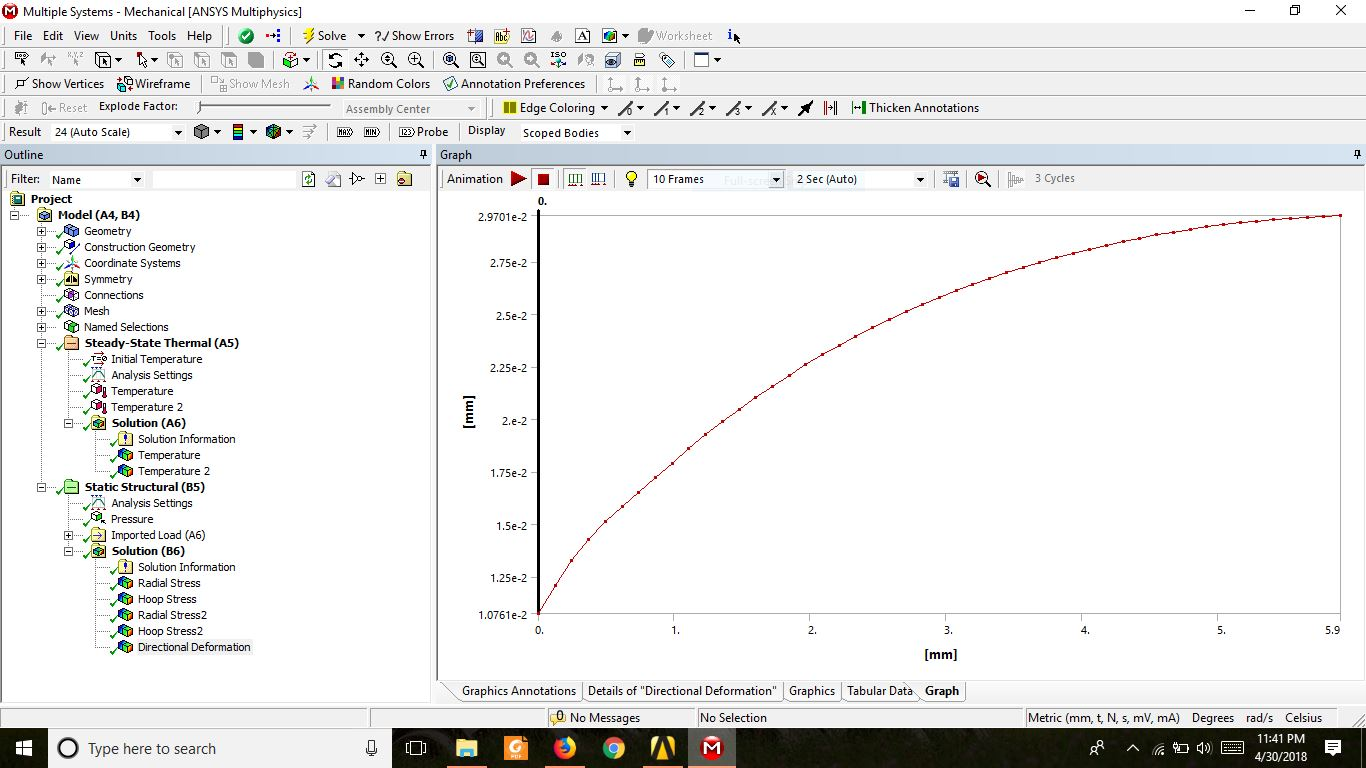•peteroznewman
Subscriber

In Excel, insert column B and use a formula to divide column A by 5.9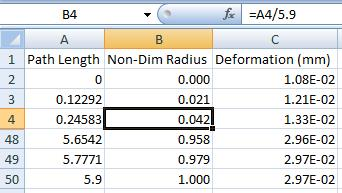Forget about the data curving up or down a little bit, the magnitude is wrong by a factor of 70.

•Md Ahad
Subscriber

Thank you very much.

And i am studying what you told me to study.I will let you know my progress as soon as possible

Regards

•Md Ahad
Subscriber

Dear sir,

Another issue has been risen out. If i want to make my solver understood that there will be no realtive displacement between each layer then how will i do it.

I meant i am getting the displacemnet graph as non linear.If i can determine that there is no relative displacement between each layer then the result should be a non linear graph.So how can i do it?

Regards

•peteroznewman
Subscriber
•Md Ahad
Subscriber

Dear peteroznewman,

I hope you are doing well.Hope you are enjoyoing your break.Sir do you have knowledge on mathematica? All the graph i have given to you solved by mathematica. So if you do have knowledge about that you can help me knowing the mathematical model, the assumptions made by the mathematical model, the inputs to the numerical model, the time-history of the materials in the numerical model.I will give you all the information to help me learning that sir.

Thanking you.

•Md Ahad
Subscriber

Dear peteroznewman,

Sir i have changed the model to get a similar graph on mathematica. I have used mixture rule for the middle layer .Here you will get all the details about the model. I am attaching a pdf of the program. If you help me showing the video that how can i make this model on workbench(mechanical) .I will be grateful to you learning that.

Regards

•Md Ahad
Subscriber

Sir

Have you understood the model sir?

Regards

•peteroznewman
Subscriber

I had a first look at the code. Who wrote the code? There is often documentation that goes along with code. Is there any other supporting documentation for this code?

A conductivity material property, K2, is a function of radius:

K1 = 2.0; K3 = 11.7; (*watt/m^2c*)

K2[r_] := 1/(r2 - r3)*((K1 - K3)*r + (r2*K3 - r3*K1));

(*K1=2.0;*)

(*K2=K1;*)

(*K3=K1;*)

But commented out is a set of statements where K1, K2 and K3 all equal 2.0.

Is your goal to build an ANSYS model that reproduces the variable K2 as a function of radius?

Or is your goal to build an ANSYS model that reproduces the mathematica output when all values of K are equal to 2.0?

If you need conductivity as a function of radius, then you will have to implement Field Variables in ANSYS. Look at section 9.2 in the ANSYS Help.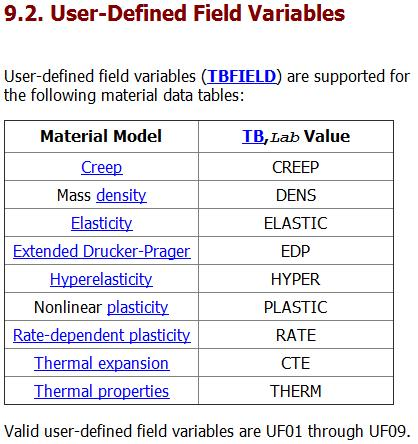•Md Ahad
Subscriber

sir

The k2 of the model that means (k2)the middle layer of the model will be the variable of the radius.

Don't consider the commented portion sir. Sir can you help me showing the video of this model in ansys? As am i very new in ansys so i could have cleared my conceptions regarding this model sir.

Regards

•peteroznewman
Subscriber

I have to learn how to create a material with a Field Variable, which will take some time.

In the mean time, you can see if you can get an ANSYS model to agree with the output of this program when all the material properties are a constant by commenting out the material variable properties for the second layer: K2, Alpha2, Nu2, and E2 and uncommenting the constant values for those properties.

What I have learned by reading the code is that it is using linear elastic material properties and there is no plasticity in the model.

I assume the radius values are in mm, and this code sets r1 = 226. The ANSYS models you provided had r1 = 4.1 mm. Make the mathematica code r1 = 4.1 to match this ANSYS model. Then the mathematica equations give us: r2 = 4.7, r3 = 5 and r4 = 6.6.  You provided a model called sss.wbpj that had three layers, but that model had r4 = 10.  Make mathematica Lstlayt = 5.0 to match this ANSYS geometry.

I know how to change 2D ANSYS models between Plane Stress and Plane Strain and the value of stress is different between those settings. You can look up the engineering equations that calculate stress for those two assumptions in a solid mechanics reference (book or Internet), and compare what you find with the Stress Calculation code in mathematica then show me in your reply which assumption has been programmed into mathematica: Plane Stress or Plane Strain.

•Md Ahad
Subscriber

Sir

First i thought that i will make a model that will have 3 layers and the middle of the layer will provide some more layers that will hold the properties of the such as k2,alpha2 etc of the average of the 1st and 3rd layer.So what i did i just drew a linear line from the first and third layers and find out the properties of the middle layers from the line by plotting distance on the x axis and properties on the y axis.Because i didn't know whether ansys can make the middle layer according to the variable properties by radius or not.

1. Sir i will study according to your suggestion.But sir as i don't know how to make middle layer following the properties by the mixture rule i used in mathematica. Sir i want to make the model according to the code i gave you of the mathematica. So sir if you make a model of the picture i have attached now. I will study harder and find out new conclusions.

2.Sir you just use plane strain in your stress and deformation calculation.Further i will change it to plane stress if needed as you had learned me earlier.I hope the result will be just like the graph i had given to you earlier.Sir if you have any doubt, try to get help from the code of the mathematica. Sir try to give the video of how you make the model. I easily get the concepts then as i am still a beginner sir.

3.Sir as i am your sincere student.I will study whatever problems you face making my model and getting the similar result from that model and then i will try to solve your doubts.And you know, i have learnt all the things you have tried to deliver . I am continuing my work on the mechanical now.. Thank you very much for all your support so far.

•peteroznewman
Subscriber

Because i didn't know whether ansys can make the middle layer according to the variable properties by radius or not.

Yes it can, you have to create a material with a field variable as described above.

2.Sir you just use plane strain in your stress and deformation calculation.Further i will change it to plane stress if needed as you had learned me earlier.I hope the result will be just like the graph i had given to you earlier.Sir if you have any doubt, try to get help from the code of the mathematica.

I looked at the code some more, it is written in terms of r and theta, therefore this is an axisymmetric model. What I find troubling is that there are no equations in terms of the axial direction, which might be called z (or y or x). Yet axisymmetric models have to satisfy equilibrium in all three directions. Axisymmetric equations of equilibrium are different from 2D Plane Stress and 2D Plane Strain, so forget my request to show me those equations. You should go look for a reference on the equilibrium equations for axisymmetric models and show me those equations, then compare them to the mathematica code.

•Md Ahad
Subscriber

Sir

You can make 2D plane strain model. Forget this portion axisymmetric for r and theta. We just make this model just like before.I hope the result will be satisfactory.

Sir what can be done with the problem second layer? Can you help me studying out for a solution for this layer?

Regards

•peteroznewman
Subscriber

You have two "black boxes" that take inputs and produce outputs. One is an ANSYS model, the other is Mathematica code that is also a model.

You can give each black box the same inputs and if they each generate the same outputs, you can say that they perform the same function inside the black box.

But what do you do when they give different outputs for the same inputs? Either there is a mistake in one model or there is no mistake, but one black box actually does not perform the same function as the other black box. For example, the ANSYS model may be built to evaluate 2D plane strain but the Mathematica model may be written to evaluate axisymmetric models.

I suggest we first find out if the two models produce the same outputs for the same inputs.  That means making K2, Alpha2, Nu2, and E2 all constants. Then we can quickly generate outputs from both ANSYS and Mathematica.  If the outputs are identical, then we can go to the next step of learning how to use Field Variables in ANSYS.  But if the outputs are different, then we have to study what is inside the black box and see what the Mathematica code is doing.

•Md Ahad
Subscriber

Ok sir .

I have got you.Then SIr as you have told , i am trying to study about if the 3 layers are homogeneous then what will be the output in mathematica. And sir you try to make the model as i told in my last message.

Sir i will have no problem if the outputs are different because of the solver of ansys and matheatica. But i will not be satisfied if i make the wrong model of how the model is told on the mathematica. I am trying to compare results of the same model between the simulated on ansys and numerical solution from mathematica.

And ok Sir , i am studying of what you have told me to do.

Thanking you

•peteroznewman
Subscriber

And sir you try to make the model as i told in my last message.

You make the ANSYS model and send me the archive and the Mathematica code that matches the constant material properties in the ANSYS model. I will check if the inputs match. Send the Mathematica output. I will check if the outputs match. You also compare the outputs, are they the same or different?

Sir i will have no problem if the outputs are different because of the solver of ansys and mathematica.

I will have a problem if the outputs are significantly different. There is no point implementing variable material properties for layer 2 in ANSYS if the outputs don't match.

•Md Ahad
Subscriber

Ok Sir

I am trying to do as you have told.But sir how can i make the second layer on my model as i dont know how to define material properties on the second layer by the mixture rule i applied on the mathematica.

So what can i do now as you have taught me earlier how to make layers, i can make some layers in the second layer and on those layer i can vary the material properties linearly by radius. I think this wont give so many difference on the results comparing with mathematica graph. And sir i am giving you the update of mathematica results for the homogeneous cylinder layers(2D plane strain) that will help us to understand the difference of the results from the mathematica model with ansys model.

profound Regards

•peteroznewman
Subscriber

Attached is Mathematica code for a single material with the following properties:

Young's Modulus 10000 MPa
Poisson's Ratio 0.25
Thermal Conductivity 2.0 W/m/C
Thermal Expansion 1.1e-6

The geometry is for an inside radius 226 mm and outside radius 227.5 mm.

The boundary conditions are 1000 C inside and 950 C outside with a 10 MPa inside pressure.

Make an ANSYS model to these specifications and run the attached Mathematica code and see if the output agrees.

•Md Ahad
Subscriber

Sir

Due to some personal problems, i am lately giving the reply. I will be in my previous flow after couple of days. I have completed the model you gave previously in workbench. I am attaching the archive file with the results of temperature and stress vs distance picture too.

Profound Regards

•peteroznewman
Subscriber

Thank you for the Archive. I noticed is there is only one element through the thickness. The elements used are linear PLANE183 elements, so with only one element, the stress profile through the thickness can only be a straight line. In this particular model, that is the exact solution, so you got away with one element, but in general, you almost always want a minimum of two elements through the thickness to detect curvature in the result.

I checked all the inputs to the ANSYS model, and all is correct except you selected Plane Stress and you said you wanted Plane Strain.  ANSYS won't let you change to that for the SS Thermal. To accomplish that for the Static Structural, you need to connect just the SS Thermal to the Setup cell of the Static Structural, then you can select Plane Strain.  In this particular case, the radial normal stress (x-direction) component is the same for both assumptions, but the axial normal stress (z-direction) is zero in the Plane Stress assumption but it is 466 MPa for the Plane Strain assumption. However, Mathematica is not calculating this component of stress.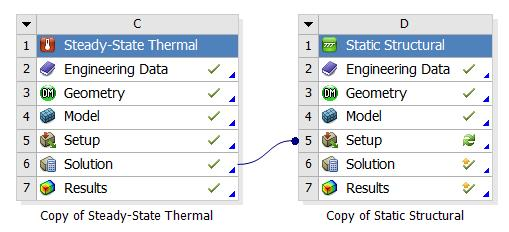I assume the graphs above are from Mathematica.  If so, then they agree with the ANSYS results.

You are ready to advance to the next phase of testing the agreement. Build a 3-layer ANSYS model with 3 different constant material properties and see if Mathematica and ANSYS still give the same result.

•Md Ahad
Subscriber

Sir

that graph is the result of the archive file.That was not mathematica result.I will reply mathematica result (graph) as soon as possible. Since um in a trouble, i cant continue my work with the regular flow.Yes sir, i made mistake that i worked on plane stress. That would be plane strain. I will correct next time and reply will all as you have told.

Thanks Sir